Thank you for visiting nature.com. You are using a browser version with limited support for CSS. To obtain the best experience, we recommend you use a more up to date browser (or turn off compatibility mode in Internet Explorer). In the meantime, to ensure continued support, we are displaying the site without styles and JavaScript.

# Topological sound in active-liquid metamaterials

## Abstract

Liquids composed of self-propelled particles have been experimentally realized using molecular, colloidal or macroscopic constituents1,2,3,4,5. These active liquids can flow spontaneously even in the absence of an external drive6,7,8. Unlike spontaneous active flow9,10, the propagation of density waves in confined active liquids is not well explored. Here, we exploit a mapping between density waves on top of a chiral flow and electrons in a synthetic gauge field11,12 to lay out design principles for artificial structures termed topological active metamaterials. We design metamaterials that break time-reversal symmetry using lattices composed of annular channels filled with a spontaneously flowing active liquid. Such active metamaterials support topologically protected sound modes that propagate unidirectionally, without backscattering, along either sample edges or domain walls and despite overdamped particle dynamics. Our work illustrates how parity-symmetry breaking in metamaterial structure combined with microscopic irreversibility of active matter leads to novel functionalities that cannot be achieved using only passive materials.

## Main

We design active metamaterials with transport properties akin to those of quantum Hall fluids13 by confining active liquids in periodic geometries that generate gapped density-wave spectra. Recent studies of topological acoustics have revealed that spectral bands characterized by topological invariants host (in their spectral gaps) robust mechanical states14,15,16 and sound modes that propagate unidirectionally along sample edges and interfaces11,12,17,18,19,20,21,22. However, the translation of topological-acoustic designs from macroscopic prototypes to soft materials has so far proved challenging, because overdamped particle dynamics overcome inertia and suppress the propagation of ordinary sound waves at the microscale. To address this challenge, we elucidate the relationship between emergent active flow and the spectrum of topological density waves in a confined liquid composed of self-propelled particles that have overdamped dynamics and align their velocities, that is, a confined polar active liquid.

To obtain generic results, we use a continuum-mechanics description of polar active flow. The analogue of Navier–Stokes equations that describes a one-component fluid of self-propelled particles (with overdamped particle dynamics, see Methods) is the Toner–Tu equations6,8,23, which in their simplest form read: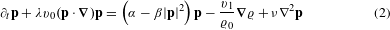where ϱ(r, t) is the density of active particles that fluctuates around its mean value ϱ0. The polarization field of the material, p(r, t), denotes the local average orientation of the velocities of the self-propelled units, which, when isolated, all move at the same speed v0. The effective viscosity, ν, the diffusivity, Dρ, and the other (positive) hydrodynamic coefficients λ, v1, α and β have been computed from a number of microscopic models in refs 2,24,25,26,27; α and β are the Landau coefficients used to model the spontaneous breaking of rotational symmetry; v1 relates pressure and density. In the Methods, we provide a concise introduction to the Toner–Tu model and explain how the left-hand side of equation (2) originates from overdamped dynamics of p and not from momentum conservation.

Numerically solving equations (1) and (2) in the connected-annuli geometry of Fig. 1a, we find the emergence of a uniform steady chiral flow in each annulus. As this flow is a consequence of spontaneous symmetry breaking, left-handed and right-handed orientations are equally likely to occur (provided no explicit symmetry breaking occurs, see for example, ref. 28). These general continuum-mechanics results are confirmed by particle-velocity maps measured from a prototypical microscopic model shown in Fig. 1b (see Supplementary Information). As particle velocities align in the region shared between two adjacent annuli, the fluid within these annuli circulates in opposite directions, in analogy with either engaged counter-rotating gears or antiferromagnetic spins. Similar behaviour of confined active fluids29,30,31,32 was observed in bacterial fluids experiments9 and simulations of agent-based models33.

When a homogeneous polar liquid flows through interconnected annuli, the channel geometry determines the mean polarization p0(r), which is proportional to the steady-state velocity field. We now elucidate how this emergent spontaneous flow impacts sound propagation. We linearize equations (1) and (2) in the limit in which confinement suppresses fluctuations in the direction transverse to the mean flow and deep in the polar liquid phase, in which case both α and β are only weakly dependent on ρ. We define π(r, t) = p(r, t) − p0(r) and ρ(r, t) = ϱ(r, t) − ϱ0, and confirm that density waves propagate over a finite range of wavenumbers q: |α| /c q c/(ν + Dρ), wheresets the magnitude of the speed of sound (see refs 6,8 and Methods). In this regime, density fluctuations obey a wave equation that depends on p0:Whereas (acoustic) density waves in simple driven fluids11,12 arise only in systems with inertial dynamics, such waves in polar active liquids survive even in the overdamped limit. In polar active fluids, these waves originate from retardation effects in the coupling between the velocity and polarization fields (see ref. 8 and Methods). Figure 2a shows the dispersion relation of density waves for a homogeneous polar liquid uniformly flowing along the x-direction (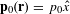). Note that the speed of sound depends on the orientation of the wavevector q relative to p0, because Galilean invariance is broken in equation (2).Figure 2: Dispersion of density waves in active metamaterials either with or without topological edge states.

Our design of topological metamaterials exploits both microscopic irreversibility induced by activity and parity-symmetry breaking of the structure. To highlight how the interplay between activity and structural design leads to metamaterials that globally break time-reversal symmetry, we contrast two simple geometries of interconnected channels: one based on the square lattice (Fig. 2b), and one based on the Lieb lattice (Fig. 2c). Solving equation (3) numerically in a square-lattice geometry (see Methods), we show that the wave spectrum contains degeneracies at the edge of the Brillouin zone where two spectral branches intersect (point M). Note that the corresponding steady-state flow is invariant with respect to simultaneously inverting the arrow of time and performing a lattice translation. By contrast, the degeneracy at point M is lifted for the Lieb lattice and a gap opens. Unlike the square lattice, the Lieb lattice has an odd number of rings per unit cell and, therefore, a net circulation of steady-state flow. Heuristically, the spectral-gap opening stems from the frequency difference between density waves propagating along versus opposite to flow with a non-vanishing net circulation. As a result, a gap opens only for unit cells that are chiral. In the limit v0p0/c 1, we rewrite equation (3) as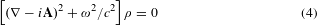where Aω(λ + 1)v0p0/(2c2), and note that the steady-state velocity field v0p0 couples minimally to the wavenumber of the density wave11. The emergent chiral flow plays the role of a synthetic gauge field for a charged quantum particle, whereas its curl, the vorticity, acts analogously to a magnetic field that lifts spectral degeneracies.

We establish the topological nature of the band structure corresponding to equation (3) in the Lieb lattice by calculating (for each band) an integer-valued topological invariant called the Chern number, Cn (see Methods and ref. 13 for an introduction). For almost all of the bands in the spectrum, and for a wide range of values of the mean polarization p0, we find that Cn ≠ 0 (Fig. 2c, d). As Cn is an integer, it cannot vary smoothly from within the sample to the exterior (where Cn = 0). Therefore, Cn can change only if the bandgap closes along the sample edge, locally enabling edge-mode propagation13. Such edge modes, shown in Fig. 2d, are called topologically protected because they arise from the presence of topological invariants in the bulk, irrespectively of the sample’s shape, disorder or strong fluctuations due to activity. As in quantum Hall fluids, the topological edge modes are chiral, that is, they propagate along a single direction, as can be seen in the Supplementary Movie. The chirality of the edge modes reflects the chirality of the flow within the unit cell; that is, if the flow is reversed so is the direction of mode propagation, as the movie shows. The system edge acts as a robust acoustic diode—topological density waves, unlike ordinary waves, propagate unidirectionally along the boundary and do not backscatter even if obstacles or sharp corners are introduced, as demonstrated in Fig. 3a.

Similarly, along the boundary between two regions of distinct flow chirality, Cn varies from one integer value to another. Therefore, in this region of space, the bandgap must vanish, which leads to the existence of topologically protected waves along this interface. A topological waveguide can be sculpted in the bulk by deleting a row of annuli, as in Fig. 3b. For this sample, topologically robust density waves propagate through the irregularly shaped domain wall in the bulk of the metamaterial. However, if the domain wall has both a row deletion and a half-column displacement, then the chirality of flow does not change across the wall. Consequently, modes associated with the domain wall are not topologically protected and do backscatter in the bulk, as exemplified in Fig. 3c.

Whereas the existence of edge waves in polar active liquids is topologically protected, their penetration depth into the bulk can be tuned by changing the flow speed. As shown in the Methods, by considering the minimal coupling form of equation (4) relevant to the motion of density waves in the limit v0p0/c 1, we expect the penetration to be exponential with a penetration depth scaling as |A| −1 ac/(v0p0), where a is the lattice spacing. We stress that this spatial structure differs from the Gaussian profiles of quantum Hall states that share similar topological properties. These predictions are in good agreement with the numerical resolution of the full equations of motion: as shown in Fig. 3d, the penetration of the edge modes is exponential and decreases with the mean-flow speed.

Having explored the phenomenology of chiral states in confined active liquids, we can now compare this realization of a topological metamaterial with those achieved in driven liquids11,12. First, in both cases, to achieve a small penetration depth it is necessary that the speed of flow be appreciable relative to the speed of sound. For a simple fluid, this is a limitation—driving the fluid at speeds near the speed of sound leads to flow instabilities either in the bulk or in the boundary layer of the fluid. By contrast, for active liquids, the speed of flow v0p0 and the speed of sound c are distinct parameters entering the hydrodynamic equation (2) and may, in general, be comparable, so that the chiral edge state may be readily observable. Second, whereas metamaterials composed of driven fluids require motors at each component to provide the drive, for an active liquid the drive is provided by the particles composing the liquid, whereas the confining channels prescribe the emergent chiral flow. Third, topological density waves in polar active liquids originate from Goldstone modes due to broken rotational symmetry. As a consequence, they can propagate even if particle dynamics are overdamped—paving the way towards colloidal and other soft-matter realizations of mechanical topological insulators.

We examined topological sound in metamaterials based on polar active liquids, but our approach can be applied to wave propagation in other time-reversal-symmetry-broken active systems. Our results epitomize the defining feature of topological active metamaterials: they combine the microscopic irreversibility inherent in active matter with structural design to achieve functionalities absent in passive materials.

## Methods

The Methods is organized as follows. We first provide a derivation of the Toner–Tu equations23 introduced in the main text by building on conservation laws and symmetry principles. We also recall how density waves can propagate in polar active fluids despite overdamped microscopic dynamics. We then establish a rigorous analogy between the effect of spontaneous active flow on density waves and the effect of a gauge field on the wavefunction of a charged quantum particle. We show how a scaling analysis of the equation for density waves leads to an estimate for the penetration depth of a topological edge state in an active metamaterial. Finally, we include discussions of the calculation of Chern numbers and of COMSOL simulations.

### Toner–Tu hydrodynamics of polar active liquids.

The hydrodynamic equations of a passive polar liquid take into account three slow variables: the usual density, ρ(r, t), and velocity, v(r, t) fields, as well as a broken-symmetry field, the polarization p(r), defined as the local average of the particle orientations. When the polar units that form the liquid propel themselves on a solid surface, momentum is no longer conserved, because the substrate acts as a momentum sink. Such systems are referred to as dry active matter, even though the particles may propel in a fluid medium as in, for example, refs 1,2. The substrate enables preferential alignment of the particles’ velocities with their polar orientation. The hydrodynamic equations of the resulting polar active liquid read: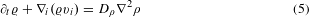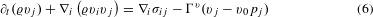where we have introduced the symmetric part of the strain-rate tensor vji ≡ (1/2)(jvi + ivj) and the vorticity tensor ωji ≡ (1/2)(jviivj). Note that the components of the velocity vector vi for this one-fluid model are the coarse-grained velocities of the self-propelled particles composing the active liquid and not of the potential surrounding fluid (for example, air or solvent). The first (continuity) equation reflects mass conservation and includes a diffusive term Dρ2ρ. The second equation includes the liquid stress tensor σ as well as an active frictional term proportional to Γv. This term differentiates equation (6) from the usual Navier–Stokes equations as it explicitly breaks momentum conservation. For the sake of simplicity, we consider here a linear coupling between the velocity and the polarization (the hydrodynamic coefficient v0 has the dimensions of a speed and scales with the speed of an isolated active particle). Equation (7) describes the relaxational dynamics of the polarization (see ref. 6). The left-hand side of equation (7) contains the comoving (second term), corotational (third term) time derivative of the polarization. The right-hand side of equation (7) includes the effective Hamiltonianand the dissipative coefficient Γp along with two frictional terms. The first frictional term in equation (7) contains the friction coefficient ν3 and describes the friction between particle and substrate—this term is responsible for the ‘weathercock effect’; that is, the polar particles’ local alignment with the flow (see, for example, refs 5,34). The second friction term in equation (7) contains the friction coefficient ν2 and originates from the friction between an individual polar particle and the surrounding active fluid (itself composed of polar particles). The sign and the magnitude of ν2 controls the strength of alignment of the particle polarization with the local elongation (or compression) axis of the flow.

We can also consider equations (5), (6) and (7) in the limit for which the frictional Γv term dominates equation (6). In this overdamped limit, equation (6) reduces to a constraint equation, v = v0p, and the hydrodynamics is fully captured by mass conservation and the dissipative dynamics of the polarization field. A gradient expansion of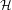then yields8,23: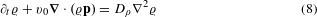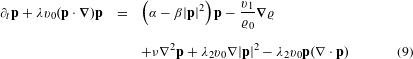where all of the hydrodynamic coefficients depend a priori on the local density. Note that whereas for a system with Galilean invariance, λ = 1 and λ2 = 0, for the polar active liquid, which lacks this symmetry, these parameters may be arbitrary. Studies of realistic microscopic models have found λ to be positive, less than, and of order 1, and for the numerical computations performed in this work, we assume λ = 0.8 (refs 2,24,25). The lack of Galilean invariance as well as momentum conservation leads to the α and β terms in equation (9), which suggests a preference for either zero or nonzero velocity—depending on the sign of α. In the letter, for the sake of simplicity we focus on the case in which the λ2 terms are negligible. In this case, equations (8) and (9) reduce to equations (1) and (2) in the main text, and are reproduced here:equations (10) and (11) are sufficient to capture the phenomena associated with linear density waves in a polar active liquid relevant to our analysis. In that limit, the polarization field itself defines the fluid velocity, so that the coupling between the polarization field and the density gradient has an effect analogous to that of a pressure gradient in an equilibrium liquid.

### Linear density waves for Toner–Tu liquids.

For the case α > 0, the Toner–Tu equations result in a steady state of the fluid with spontaneous flow in the bulk, such that p02 ≡ |p0| 2 = α/β. Although in the bulk, the spontaneous flow direction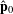could be arbitrary, in physical realizations of active liquids, the boundaries fix. For example, in an open channel,is parallel to the channel walls. In the Lieb-lattice geometry, we have solved equations (10) and (11) for a sufficient time for the dynamics to relax to a steady state. We find that this steady state, plotted in Fig. 1a (also see Supplementary Fig. 2), has the features of the spatial profile observed from our particle-based simulations, although the particle-based simulations lead to a smoother profile (Fig. 1b).

In the analysis performed for the density-wave computations, we take a particularly simple form of the steady state, based on the profile we observe. We postulate the polarization has magnitude unity everywhere and is oriented azimuthally, that is, perpendicular to the vector connecting the position of the fluid to the nearest annulus centre. In the regions of overlap between annuli, we linearly interpolate between the two annular flow profiles. This spatial profile is plotted in larger samples in Fig. 3b, c of the main text.

Thus, given a spontaneous steady-state flow field p0, we expand π(r, t) = p(r, t) − p0(r) and ρ(r, t) = ϱ(r, t) − ϱ0 to find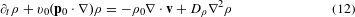Whereas in simple fluids sound propagates as a result of inertia, in polar active liquids the role analogous to inertia is played by the retardation effects in the coupling between the velocity and polarization fields. For the case of propagating waves, the right-hand side can be decomposed as the sum of a dominant anti-Hermitian matrix that governs wave dispersion and a perturbatively small Hermitian matrix that governs wave attenuation. As we are interested in the behaviour of an active fluid deep in the ordered phase, we have assumed α to be constant. The ρ dependence of α, which leads to additional dissipative terms, would be most significant near the phase transition from an isotropic to a flowing steady state. There are two notable differences for the propagation of density waves in an active liquid compared with a simple fluid: the α term acts as an additional dissipative term for sound in an active liquid; and one of the convection terms contains the coefficient λ (≠ 1). Due to this second difference, the equation of motion can no longer be ‘Galilean boosted’ into a different reference frame by replacing the laboratory-frame derivative t by a convective derivative.

To closely examine the mode structure in equation (12), we split the vector v into components v and v, respectively parallel and perpendicular to. Having in mind the flow of active liquid inside a narrow channel, we assume that confinement strongly suppresses fluctuations in the transverse direction and therefore assume that the density waves propagate only along the channel direction. We therefore ignore the derivatives of p and ρ along the direction perpendicular to. Under this assumption, equations (12) reduce to: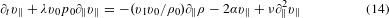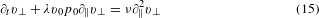Let us now consider equations (13) and (14) for the density and the longitudinal velocity modes in an active liquid. We can calculate the dispersion relation ω(q) = ω′(q) + ′′(q) for density waves in active liquids in the limit q c/(Dρ + ν), where q is the wavenumber of the density wave:Two comments are in order. Firstly, due to spontaneous flow, the sound-wave frequency generically has a real component, which is plotted in Fig. 1a of the main text, and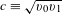is the speed of sound. We also note that in the considered channel geometry the active fluid has a single sound mode unlike unconfined polar liquids23. Secondly, in the limits |α| /c q c/(Dρ + ν), the quality factor Q = ω′/ω ′′ c/(Dρ + ν)q is always much larger that one; therefore, waves can almost freely propagate over a number of unit cells before being damped by diffusion and orientational elasticity. A candidate for a possible experimental realization could be colloidal rollers confined to microchannels2. For this system the elastic constant is about ν 10−9 m2 s−1, and translational diffusion is subdominant. Taking a speed of sound of the order of the speed of the motile bodies c 10−3 m s−1, and q 103 m−1 at the edge of the Brillouin zone (which correspond to annuli of diameter 1 mm), we find Q 103. Confined density waves should almost freely propagate.

### Analogy with Schrödinger equation.

Note that when the dissipative components of the density-wave equation can be neglected, equation (12) may be recast as a single wave equation. By applying the convective derivative t + λv0(p0 ) to the continuity equation, equation (13), and then substituting the velocity equation of motion, equation (14), one obtains: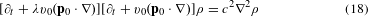The eigenvalue problem for the above wave equation has solutions in terms of the frequency ω of a time-dependent oscillation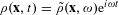. The corresponding equation has the form, provided that Mv0p0/c 1,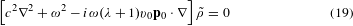or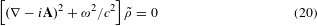where Aω(λ + 1)v0p0/(2c2). This shows that the velocity field v0p0 acts as an effective vector potential for the propagation of density waves.

### Scaling argument for penetration depth.

From the form of equation (20), we can deduce the following scaling argument for the penetration depth of a topological edge state in the relevant limit v0p0/c 1. Consider the first term, (i A)2, which shows the minimal coupling between density gradients and spontaneous flow11. The penetration depth is a length scale that originates from density gradients and therefore scales as A−1. Furthermore, A v0p0ω/c2 and depends on a characteristic frequency ω1c/a, where c is the speed of sound and a is a characteristic length scale of the material, that is, the lattice spacing. In addition, A is approximately the same from one unit cell to the next. Combining these scaling relations, ac/(v0p0). The length diverges as the flow velocity goes to zero and, therefore, as the material loses its bulk bandgap.

We also note that we expect and observe topological edge states to be localized near the edge with an exponential profile (see Fig. 3d). To see why we expect equation (20) to lead to exponentially localized states, note that if we assume ρ f(x/), and the scaling law derived above for , ac/(v0p0), equation (20) predicts f ′′ f, with a dimensionless proportionality constant. An exponential profile satisfies this approximate scaling form. Such a profile is a consequence of the fact that A does not vary over length scales larger than a unit cell, an argument that relies on the metamaterial structure of the topological state. By contrast, in the quantum Hall fluid, the frequency scale depends on the field strength and A varies over large distances, which leads to both a Gaussian profile of states in a Landau level as well as a different scaling for the penetration depth35.

### Chern numbers.

We establish the topological nature of the active-liquid metamaterial by calculating (for the Lieb-lattice spectrum) an integer-valued topological invariant called the Chern number associated with each band (see ref. 13). The Chern number Cn is analogous to the Euler characteristic of a closed surface with Gaussian curvature. Using the Gauss–Bonnet theorem, we can compute Cn by integrating a curvature called the Berry curvature Bn(q) over a closed surface formed by the first Brillouin zone (which by construction is periodic in both directions):where,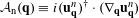is the Berry connection calculated from the uqn eigenstate of band n and wavenumber q. For our discrete data set, we use the gauge-choice-independent protocol described in ref. 36 to efficiently calculate the Chern number using a coarse discretization of the first Brillouin zone.

### COMSOL simulations.

We solve equation (3) for both a finite geometry and for a unit cell with Floquet boundary conditions (that is, periodic boundary conditions with an additional phase factor eiqx, where x is the vector connecting the corresponding parts of the two boundaries) using COMSOL Multiphysics finite-element analysis simulations on a highly refined mesh. Our simulations are a modified version of the built-in physics ‘Pressure Acoustics, Frequency Domain’ for the calculation of eigenmodes using an Eigenfrequency study and the physics ‘Pressure Acoustics, Transient’ using a Time-Dependent study for the supplementary movie. The boundary conditions are Sound Hard Boundary (Wall) everywhere except along the boundaries with periodic conditions. The equations are modified to correspond to equation (18) and entered in the Weak Form required for Finite-Element Simulations.

To obtain the dispersion relations shown in Fig. 2b, c, we perform a sweep through the wavenumbers (qx, qy) along the MΓM cut and assign the appropriate phase factors for (Floquet) boundary conditions across the unit cell. Then, we solve the corresponding eigenvalue problem at each wavenumber and plot the corresponding bands. We numerically obtain the solutions in the form of frequencies ωn(q) as well as the density eigenstates, for which the density waves are. Unless otherwise noted, to obtain good numerical accuracy, we use for the corresponding background flow a simplified model with constant |v| = p0v0 = 0.5c, pointed along the azimuthal direction of the corresponding annulus (see visualizations in insets of Fig. 3b, c). In the regions of annular overlap, we patch the flow field using an interpolation that is linear along the x-direction, and then normalize the result. For Fig. 2d, we begin with a quasi-one-dimensional lattice geometry (also see Fig. 3d), and impose a phase factor only along the periodic boundaries in the x-direction. Again, the eigenvalues are plotted, and those forming a solid region corresponding to the bulk bands are shaded in blue. For parts of Figs 2d and 3a–c, we use a finite geometry and plot a single eigenmode located in the bandgap that contains topological states. The Supplementary Movie is generated by taking frames with in-between time intervals of approximately ω−1, with many in-between time steps for the simulation, which are determined automatically by the COMSOL solver. The density excitations are initialized to 0. The source of the density excitations is chosen as the outer-most boundary of one of the annuli at the edge. The source’s angular frequency ω is chosen to be within the bandgap indicated in Fig. 2c, d, and is ≈ 0.9c/a = 0.9ω1.

### Data availability.

The data that support the plots within this paper and other findings of this study are available from the corresponding author on request.

Publisher’s note: Springer Nature remains neutral with regard to jurisdictional claims in published maps and institutional affiliations.

## References

1. 1

Schaller, V., Weber, C., Semmrich, C., Frey, E. & Bausch, A. R. Polar patterns of driven filaments. Nature 467, 73–77 (2010).

2. 2

Bricard, A., Caussin, J.-B., Desreumaux, N., Dauchot, O. & Bartolo, D. Emergence of macroscopic directed motion in populations of motile colloids. Nature 503, 95–98 (2013).

3. 3

Yan, J. et al. Reconfiguring active particles by electrostatic imbalance. Nat. Mater. 15, 1095–1099 (2016).

4. 4

Deseigne, J., Dauchot, O. & Chaté, H. Collective motion of vibrated polar disks. Phys. Rev. Lett. 105, 098001 (2010).

5. 5

Kumar, N., Soni, H., Ramaswamy, S. & Sood, A. K. Flocking at a distance in active granular matter. Nat. Commun. 5, 4688 (2014).

6. 6

Marchetti, M. C. et al. Hydrodynamics of soft active matter. Rev. Mod. Phys. 85, 1143–1189 (2013).

7. 7

Vicsek, T. & Zafeiris, A. Collective motion. Phys. Rep. 517, 71–140 (2012).

8. 8

Toner, J., Tu, Y. & Ramaswamy, S. Hydrodynamics and phases of flocks. Ann. Phys. 318, 170–244 (2005).

9. 9

Wioland, H., Woodhouse, F. G., Dunkel, J. & Goldstein, R. E. Ferromagnetic and antiferromagnetic order in bacterial vortex lattices. Nat. Phys. 12, 341–345 (2016).

10. 10

Bricard, A. et al. Emergent vortices in populations of colloidal rollers. Nat. Commun. 6, 7470 (2015).

11. 11

Yang, Z. et al. Topological acoustics. Phys. Rev. Lett. 114, 114301 (2015).

12. 12

Khanikaev, A. B., Fleury, R., Mousavi, S. H. & Alu, A. Topologically robust sound propagation in an angular-momentum-biased graphene-like resonator lattice. Nat. Commun. 6, 8260 (2015).

13. 13

Hasan, M. Z. & Kane, C. L. Colloquium: topological insulators. Rev. Mod. Phys. 82, 3045–3067 (2010).

14. 14

Kane, C. L. & Lubensky, T. C. Topological boundary modes in isostatic lattices. Nat. Phys. 10, 39–45 (2013).

15. 15

Chen, B. G., Upadhyaya, N. & Vitelli, V. Nonlinear conduction via solitons in a topological mechanical insulator. Proc. Natl Acad. Sci. USA 111, 13004–13009 (2014).

16. 16

Paulose, J., Chen, B. G. & Vitelli, V. Topological modes bound to dislocations in mechanical metamaterials. Nat. Phys. 11, 153–156 (2015).

17. 17

Prodan, E. & Prodan, C. Topological phonon modes and their role in dynamic instability of microtubules. Phys. Rev. Lett. 103, 248101 (2009).

18. 18

Nash, L. M. et al. Topological mechanics of gyroscopic metamaterials. Proc. Natl Acad. Sci. USA 112, 14495–14500 (2015).

19. 19

Susstrunk, R. & Huber, S. D. Observation of phononic helical edge states in a mechanical topological insulator. Science 349, 47–50 (2015).

20. 20

Kariyado, T. & Hatsugai, Y. Manipulation of Dirac cones in mechanical graphene. Sci. Rep. 5, 18107 (2015).

21. 21

Wang, P., Lu, L. & Bertoldi, K. Topological phononic crystals with one-way elastic edge waves. Phys. Rev. Lett. 115, 104302 (2015).

22. 22

He, C. et al. Acoustic topological insulator and robust one-way sound transport. Nat. Phys. 12, 1124–1129 (2016).

23. 23

Toner, J. & Tu, Y. Long-range order in a two-dimensional dynamical xy-model: how birds fly together. Phys. Rev. Lett. 75, 4326–4329 (1995).

24. 24

Bertin, E., Droz, M. & Gregoire, G. Boltzmann and hydrodynamic description for self-propelled particles. Phys. Rev. E 74, 022101 (2006).

25. 25

Farrell, F., Marchetti, M., Marenduzzo, D. & Tailleur, J. Pattern formation in self-propelled particles with density-dependent motility. Phys. Rev. Lett. 108, 248101 (2012).

26. 26

Solon, A. P. & Tailleur, J. Revisiting the flocking transition using active spins. Phys. Rev. Lett. 111, 078101 (2013).

27. 27

Suzuki, R., Weber, C. A., Frey, E. & Bausch, A. R. Polar pattern formation in driven filament systems requires non-binary particle collisions. Nat. Phys. 11, 839–843 (2015).

28. 28

Stenhammar, J., Wittkowski, R., Marenduzzo, D. & Cates, M. E. Light-induced self-assembly of active rectification devices. Sci. Adv. 2, e1501850 (2016).

29. 29

Woodhouse, F. G., Forrow, A., Fawcett, J. B. & Dunkel, J. Stochastic cycle selection in active flow networks. Proc. Natl Acad. Sci. USA 113, 8200–8205 (2016).

30. 30

Woodhouse, F. G. & Dunkel, J. Active matter logic for autonomous microfluidics. Nat. Commun. 8, 15169 (2017).

31. 31

Wioland, H., Lushi, E. & Goldstein, R. E. Directed collective motion of bacteria under channel confinement. New J. Phys. 18, 075002 (2016).

32. 32

Wu, K.-T. et al. Transition from turbulent to coherent flows in confined three-dimensional active fluids. Science 355, eaal1979 (2017).

33. 33

Pearce, D. J. G. & Turner, M. S. Emergent behavioural phenotypes of swarming models revealed by mimicking a frustrated anti-ferromagnet. J. R. Soc. Interface 12, 20150520 (2015).

34. 34

Brotto, T., Caussin, J.-B., Lauga, E. & Bartolo, D. Hydrodynamics of confined active fluids. Phys. Rev. Lett. 110, 038101 (2013).

35. 35

Landau, L. D. & Lifshitz, E. M. Vol. III: Quantum Mechanics, Non-Relativistic Theory (Elsevier Science, 1958).

36. 36

Fukui, T., Hatsugai, Y. & Suzuki, H. Chern numbers in discretized Brillouin zone: efficient method of computing (spin) Hall conductances. J. Phys. Soc. Jpn. 74, 1674–1677 (2005).

## Acknowledgements

We thank M. E. Cates, M. C. Marchetti, R. E. Goldstein, J. Paulose, R. Fleury, V. Cheianov, A. Abanov, Z. Yang and B. Zhang for useful discussions. A.S., B.C.v.Z. and V.V. were funded by FOM, NWO (Vidi grant), and the Delta Institute for Theoretical Physics. D.B. acknowledges support from ANR grant MiTra.

## Author information

Authors

### Contributions

A.S., D.B. and V.V. designed the project and performed the analytical calculations. A.S. and B.C.v.Z. carried out the numerical simulations. All authors contributed to writing the manuscript.

### Corresponding author

Correspondence to Vincenzo Vitelli.

## Ethics declarations

### Competing interests

The authors declare no competing financial interests.

## Supplementary information

### Supplementary information

Supplementary information (PDF 455 kb)

### Supplementary movie

Supplementary movie 1 (MP4 19553 kb)

## Rights and permissions

Reprints and Permissions

• ### Creating synthetic spaces for higher-order topological sound transport

• Hui Chen
• , Hongkuan Zhang
• , Qian Wu
• , Yu Huang
• , Huy Nguyen
• , Emil Prodan
• , Xiaoming Zhou
•  & Guoliang Huang

Nature Communications (2021)

• ### Emergent order in hydrodynamic spin lattices

• Pedro J. Sáenz
• , Giuseppe Pucci
• , Sam E. Turton
• , Alexis Goujon
• , Rodolfo R. Rosales
• , Jörn Dunkel
•  & John W. M. Bush

Nature (2021)

• ### Topology and broken Hermiticity

• Corentin Coulais
• , Romain Fleury
•  & Jasper van Wezel

Nature Physics (2021)

• ### Exceptional non-Hermitian topological edge mode and its application to active matter

• Kazuki Sone
• , Yuto Ashida
•  & Takahiro Sagawa

Nature Communications (2020)

• ### Organizing bacterial vortex lattices by periodic obstacle arrays

• Henning Reinken
• , Daiki Nishiguchi
• , Sebastian Heidenreich
• , Andrey Sokolov
• , Markus Bär
• , Sabine H. L. Klapp
•  & Igor S. Aranson

Communications Physics (2020)

## Search